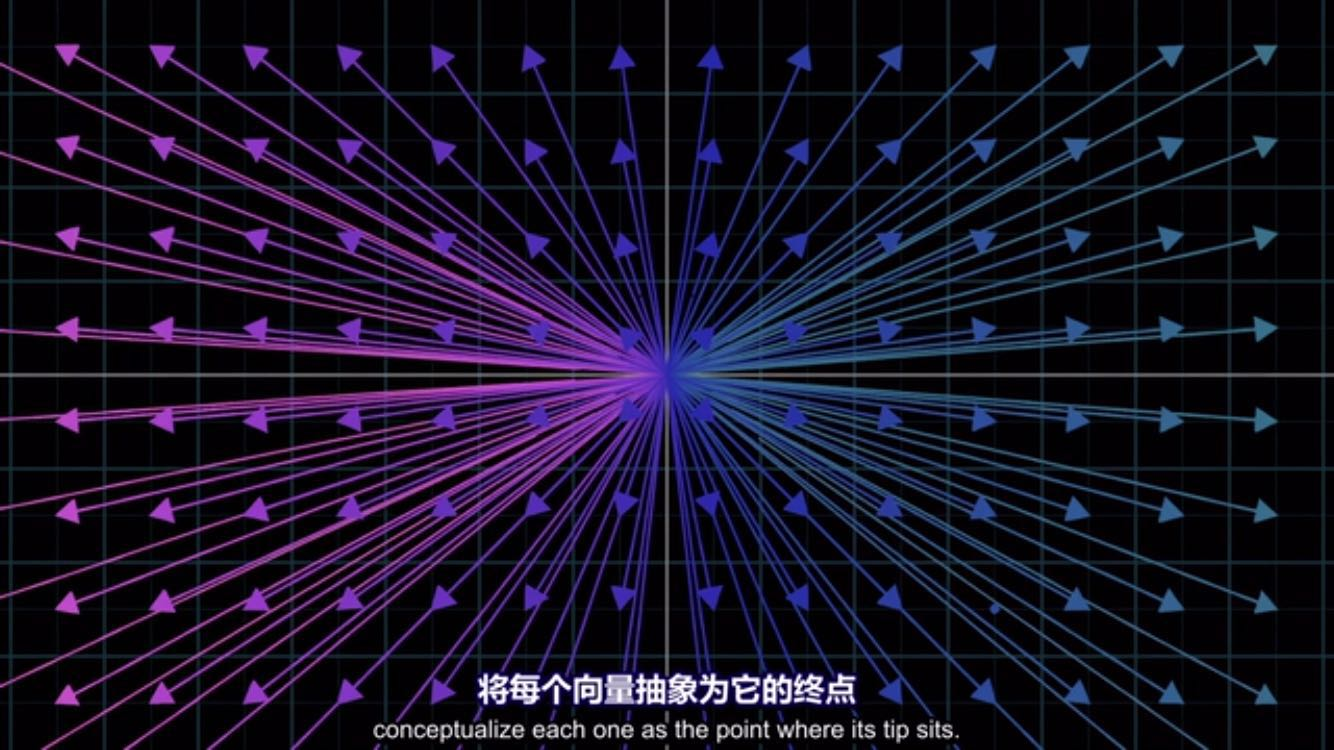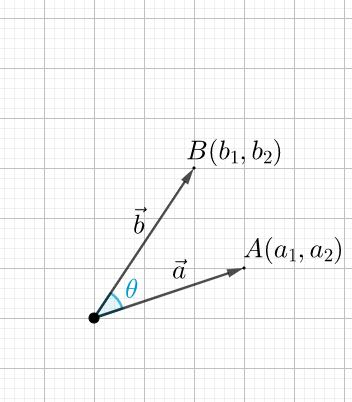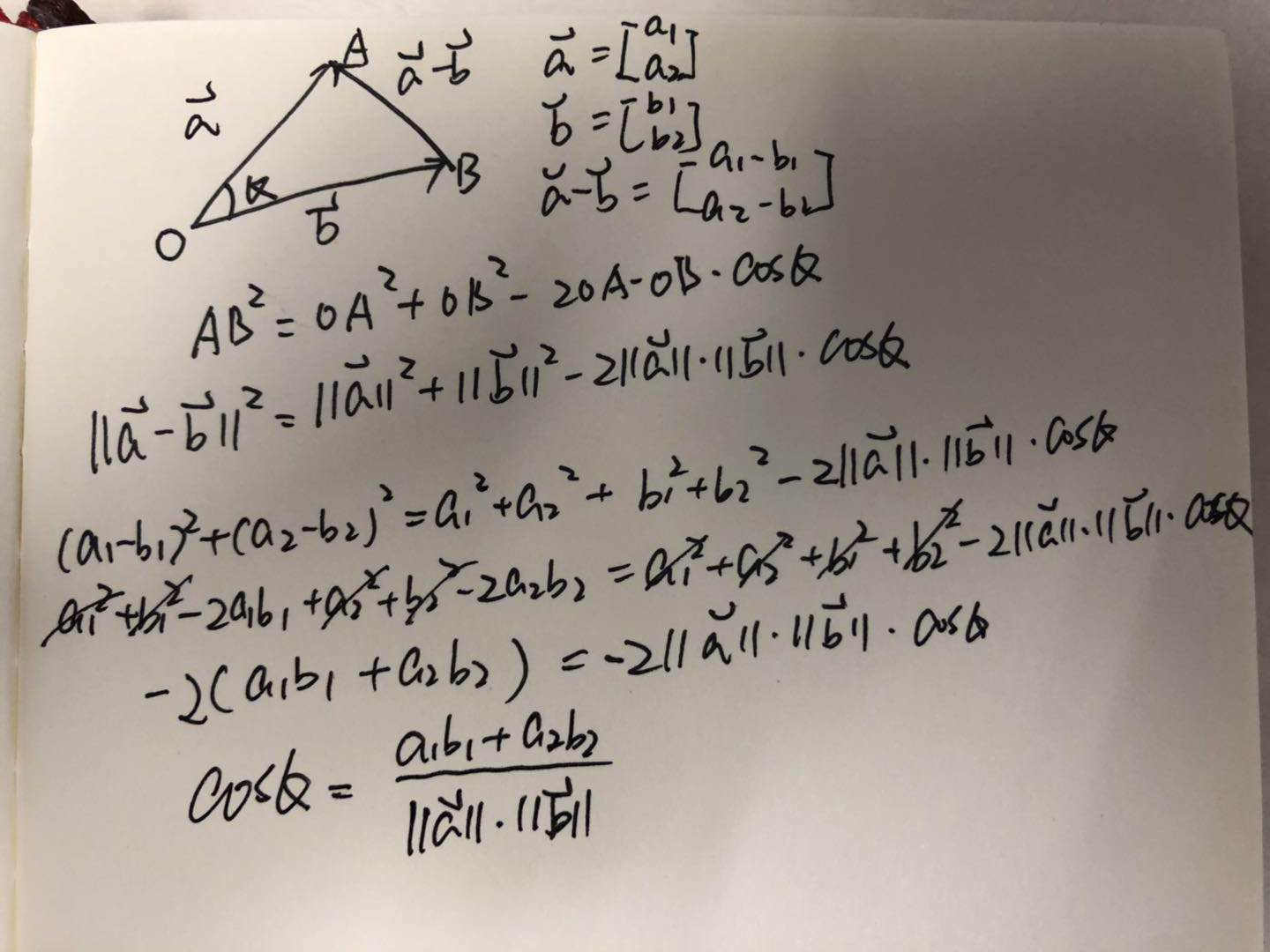# [QA] - 向量

May 04, 2018

### Q: 向量如何表示？### Q: 向量看起来就像是笛卡尔坐标系中的点，是这样吗？### Q: 同理，向量的“方向”该如何表示？$\vec{a}{\cdot}\vec{b} = a_1b_1 + a_2b_2$

${cos{\theta}} = {\dfrac{\vec{a}{\cdot}\vec{b}}{\lVert\vec{a}\rVert{\cdot}\lVert\vec{b}\rVert}}$

### Q: n纬向量指的是什么？

n个有序的数$a_1,a_2,...,a_n$所组成的数组称为n维向量，这n个数称为该向量的n个分量，第i个数$a_i$称为第i个分量。

n维向量可写成一行，也可写成一列。分别称为行向量和列向量。

### Q: 向量的运算法则是怎样的，可以加减乘除吗？

$\vec{x} + \vec{y} = \vec{y} + \vec{x}$

$\vec{x} + \vec{y} + \vec{z} = \vec{x} + (\vec{y} + \vec{z})$

### Q: 向量的点积是什么？

$\vec{x}\cdot\vec{y} = x_1y_1+...+x_ny_n = \displaystyle\sum_{i=1}^nx_iy_i$

### Q: 向量点积的几何意义是什么?

$\vec{x}\cdot\vec{y} = \lVert\vec{x}\rVert{\cdot}\lVert\vec{y}\rVert{\cdot}cos{\theta}$

$\vec{x}\cdot\vec{y} = \vec{y}\cdot\vec{x}$

$(k\vec{x})\cdot\vec{y} = k(\vec{x}\cdot\vec{y})$

$(\vec{x} + \vec{y})\cdot\vec{z} = \vec{x}\cdot\vec{z} + \vec{y}\cdot\vec{z}$

### Q: 只有三维向量有向量积吗？Hi~，i am zires, an IT engineer, live in Shanghai. You can get in touch with me via Github stackoverflow